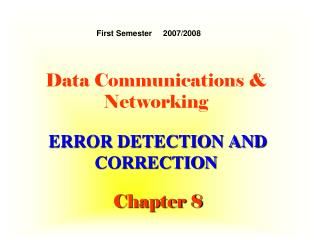Download PresentationData Communications & Networking

Data Communications & Networking - PowerPoint PPT Presentation

Data can be corrupted during transmission. For reliable communication, errors must be detected and corrected.I am the owner, or an agent authorized to act on behalf of the owner, of the copyrighted work described.
Download PresentationData Communications & Networking

Download Policy: Content on the Website is provided to you AS IS for your information and personal use and may not be sold / licensed / shared on other websites without getting consent from its author.While downloading, if for some reason you are not able to download a presentation, the publisher may have deleted the file from their server.

- - - - - - - - - - - - - - - - - - - - - - - - - - E N D - - - - - - - - - - - - - - - - - - - - - - - - - -
Presentation Transcript
1. Data Communications & NetworkingERROR DETECTION AND CORRECTIONChapter 8 First Semester 2007/2008

2. ERROR DETECTION AND CORRECTION • Types of Errors • Detection • Correction

3. Note: Data can be corrupted during transmission. For reliable communication, errors must be detected and corrected.

4. Types of Error • Single-Bit Error • Burst Error

5. Single-Bit Error Note: In a single-bit error, only one bit in the data unit has changed.

6. Burst Error Note: A burst error means that 2 or more bits in the data unit have changed.

7. ErrorDetection Redundancy Parity Check Cyclic Redundancy Check (CRC) Checksum

8. Note: Error detection uses the concept of redundancy, which means adding extra bits for detecting errors at the destination.

9. Redundancy

10. ErrorDetection methods

11. Even-parity concept

12. VERTICAL REDUNDANCY CHECK (VRC)

13. Note: In parity check, a parity bit is added to every data unit so that the total number of 1s is even (or odd for odd-parity).

14. Example 1 Suppose the sender wants to send the word world. In ASCII the five characters are coded as 1110111 1101111 1110010 1101100 1100100 The following shows the actual bits sent 11101110 11011110 11100100 11011000 11001001

15. Example 2 Now suppose the word world in Example 1 is received by the receiver without being corrupted in transmission. 11101110 11011110 11100100 11011000 11001001 The receiver counts the 1s in each character and comes up with even numbers (6, 6, 4, 4, 3). The data are accepted.

16. Example 3 Now suppose the word world in Example 1 is corrupted during transmission. 11111110 11011110 11101100 11011000 11001001 The receiver counts the 1s in each character and comes up with even and odd numbers (7, 6, 5, 4, 4). The receiver knows that the data are corrupted, discards them, and asks for retransmission.

17. Note: Simple parity check can detect all single-bit errors. It can detect burst errors only if the total number of errors in each data unit is odd.

18. 11100111 11011101 00111001 10101001 10101001 00111001 11011101 11100111 LRC 10101010 11100111 11011101 00111001 10101001 10101010 Data plus LRC LONGITUDINAL REDUNDANCY CHECK LRC

19. Two-dimensional parity

20. Figure 10.11 Two-dimensional parity-check code

21. CRC generator and checker

22. Receiver Sender T -T Data unit and checksum

23. Note: • The sender follows these steps: • The unit is divided into k sections, each of n bits. • All sections are added using one’s complement to get the sum. • The sum is complemented and becomes the checksum. • The checksum is sent with the data.

24. Note: • The receiver follows these steps: • The unit is divided into k sections, each of n bits. • All sections are added using one’s complement to get the sum. • The sum is complemented. • If the result is zero, the data are accepted: otherwise, rejected.

25. Example 4 Suppose the following block of 16 bits is to be sent using a checksum of 8 bits. 10101001 00111001 The numbers are added using one’s complement 10101001 00111001 ------------Sum 11100010 Checksum 00011101 The pattern sent is 10101001 00111001 00011101

26. Example 5 Now suppose the receiver receives the pattern sent in Example 7 and there is no error. 10101001 00111001 00011101 When the receiver adds the three sections, it will get all 1s, which, after complementing, is all 0s and shows that there is no error. 10101001 00111001 00011101 Sum 11111111 Complement 00000000means that the pattern is OK.

27. Example 6 Now suppose there is a burst error of length 5 that affects 4 bits. 1010111111111001 00011101 When the receiver adds the three sections, it gets 10101111 11111001 00011101 Partial Sum 1 11000101 Carry 1 Sum 11000110 Complement 00111001the pattern is corrupted.

28. Correction Retransmission Forward Error Correction Burst Error Correction

29. Hamming Code

30. Data and redundancy bits

31. Positions of redundancy bits in Hamming code

32. Redundancy bits calculation

33. Example of redundancy bit calculation

34. Error detection using Hamming code

35. Burst error correction example